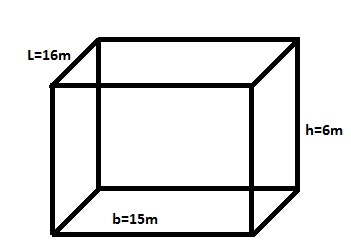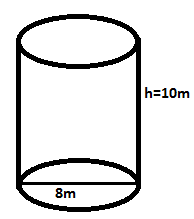# Stability -I : Chapter 1

###### SOLUTION:

Given : L =16m , B = 15m, H = 6m, RD=0.78Volume of rectangle =(LxBxH)
=16x15x6
=1440m3

We know that :
Density = Mass /Volume
0.78 =Mass/1440m3

###### SOLUTION:

Given: Diameter = 8m,

=(8/2)m
=4m
Height =10m
Mass = 400t
RD =0.9We know that:
Volume of the cylindrical tank = π2h
= 3.1416 x 4 x 4 x 10
=502.656m3

We know that:
Density = (Mass/Volume)
0.9 = 400/ (Volume of the oil)

Hence, Volume of the oil = 400/0.9
=444.44m3

Depth of oil = volume/area
Area of cylinder   =( π2r)
=(3.1416 x 4 x 4)
=502656m3

We can calculate Depth of oil = (volume of oil)/ ( area of cylinder)
= (444. 44)/(50.2656 )
=8.8418 m#### Amit Sharma

Graduated from M.E.R.I. Mumbai (Mumbai University), After a brief sailing founded this website with the idea to bring the maritime education online which must be free and available for all at all times and to find basic solutions that are of extreme importance to a seafarer by our innovative ideas.

•Pranav says:

you are right

•Harry says:

In Q9 I found ullage by subtracting
Total depth – Depth of oil

•Harry says:

Got its!!

•Arvind says:

It’s very helpful for me ..thank you sir

•Anario Nwokocha says:

Fantastic innovation

•T says:

In Q8 area of tank should be 1000m2 not 10000m2